## Puzzles and Starters

I love maths puzzles, I like solving them and I like setting them in lessons. I have written many posts about how I’ve solved them, so I thought I would put some time in and collate them into a single page for people to use as starters. Most puzzles have more than one solution, the solutions I’ve blogged are the ones I came up with. And I’ve tried to include my reasoning and other thoughts around them. Often there are more efficient and concise solutions, so I see after I’m done and some other point out. If you solve them using a different method, I’d love to hear about it, feel free to use the comments or contact me.

Note. I did try to keep this page up to a point where I could keep all the puzzles together, but it was getting far to long. I’ve left it up but you can find more by searching puzzles or puzzle.

Here is a nice number puzzle that can be used for most year groups:

A high school has a strange principal. On the first day, he has his students perform an odd opening day ceremony:

There are one thousand lockers and one thousand students in the school. The principal asks the first student to go to every locker and open it. Then he has the second student go to every second locker and close it. The third goes to every third locker and, if it is closed, he opens it, and if it is open, he closes it. The fourth student does this to every fourth locker, and so on. After the process is completed with the thousandth student, how many lockers are open?

Here is the solution to this lovely puzzle:

The numbers 2,3,12,14,15,20 and 21 may be divided into two sets so that the product of the numbers in each set is equal. What is that product? And which numbers are in each set?

Here is a nice little puzzle based on the number 71 which would be suitable for higher GCSE students and those studying A Level: 71 is the smallest prime that can be expressed as x^2 + xy + 2y^2 where x and y are nonnegative integers, find x and y giving 71.

Here is an exploration of this lovely Chessboard problem:Here is a nice triangle based puzzle from the maths challenge that could also be used for any year group:

Triangle T has sides 6,5,5 and Triangle Q has sides 8,5,5 What is the ratio Area T:Area Q?

Here is a puzzle which asked: If all the angles of a triangle (measured in degrees) are squares, what are they?” It’s from Emily Hughes’s (@ilovemathsgames) “Puzzles of the week“.

This great puzzle (solution here) is one I’d love to see on a non-calculator GCSE paper.Additional solutions can be seen here.

Another circle based puzzle is this:And a similar one:Solutions are explored here.

If it’s an easier but similar puzzle your after try this: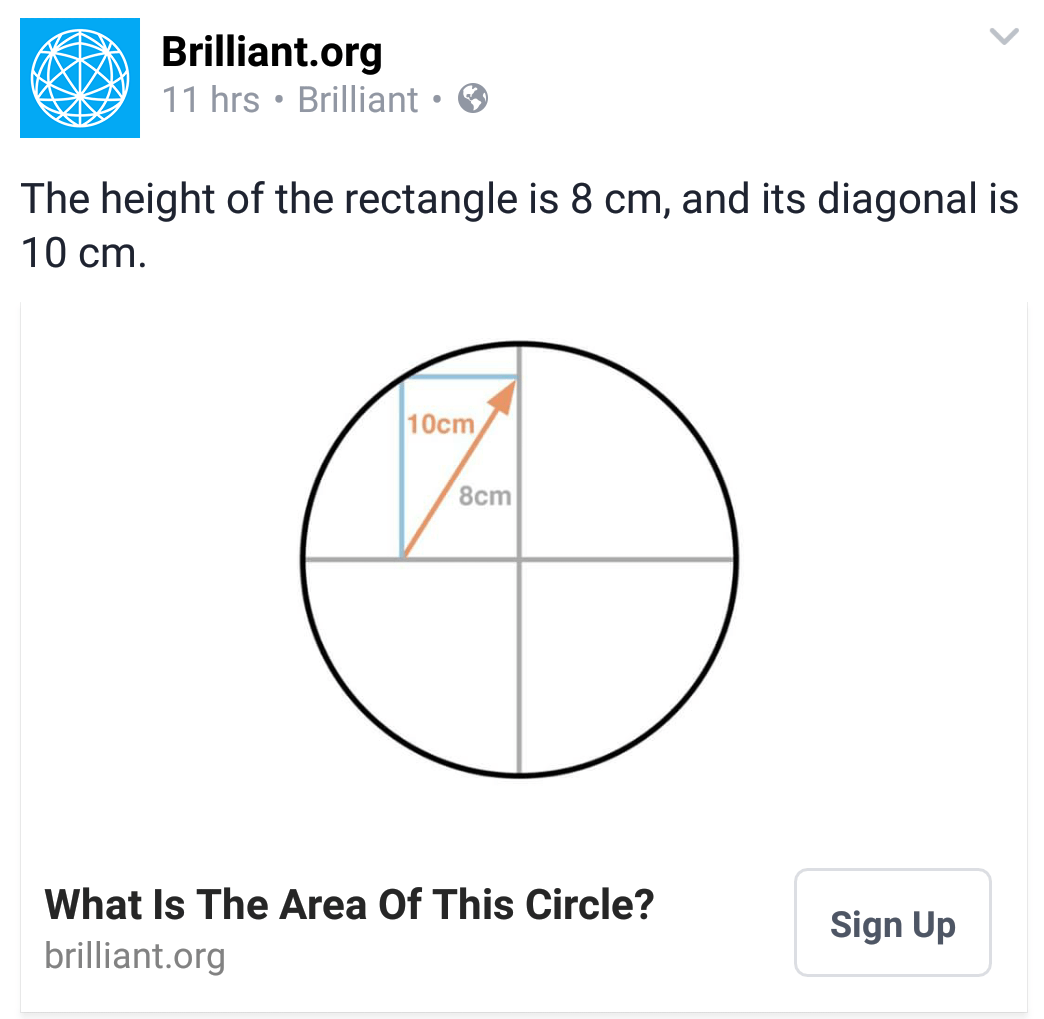This is a great puzzle based on concentric circles, that may take a little though.Here’s another lovely area puzzle from Solve my maths:Here is a look at this brilliant Quadrilateral puzzle:Here is another lovely area puzzle from solvemymaths.com: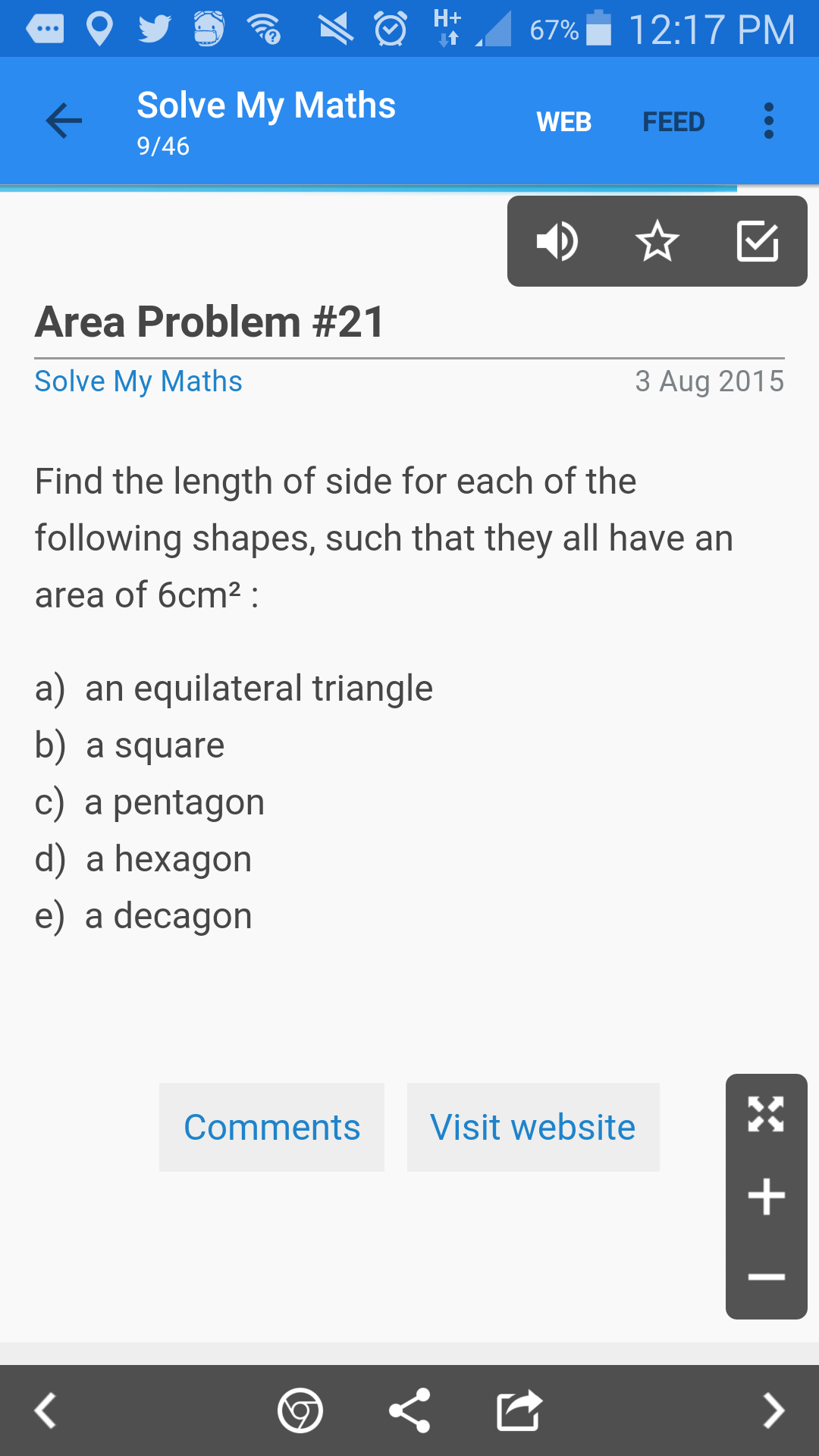This is a nice volume puzzle for higher GCSE and above:

A sphere and a conical frustrum have the same volume, the frustrum has a base radius which is twice the radius of its top. The sphere has a radius which is equal to the base radius of the frustrum. What is the ratio of the diameter of sphere to the height of the frustrum?

Here 8s an exploration of this lovely cross – curricular geography puzzle:And here is a look at this lovely trig problem!: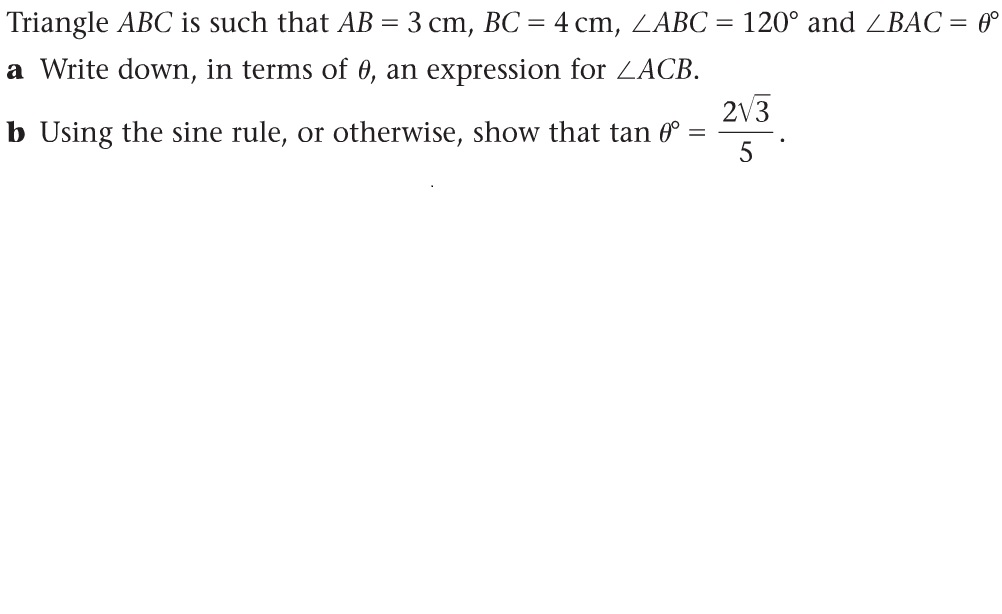Here is another lovely trigonometry based puzzle:And ANOTHER lovely trigonometry puzzle – this is probably my favourite:And this is also tricky for similar ability:

Find all solutions in non negative integers a,b to (a)^1/2 + (b)^1/2 = (2009)^1/2

This one is quite algebraic, again good for higher GCSE and/or A Level:

The sum of two squares doubled is the sum of two squares. Prove it algebraically and geometrically.

Another nice one for GCSE students maybe this lovely puzzle combining angle reasoning with algebra:Blaise of glory:This is a nice puzzle that will encourage some algebra:“If you keep two sides of a triangle the same but let the other side change, you could make an infinite number of triangles. Of all those possible triangles, which one has the largest area?”

And in keeping with this theme here is my solution to this fantastic triangle puzzle that is accessible to all age ranges and can prove a great discussion point with the various solutions available.Here is my solution to this lovely puzzle:Here we have an exploration of this lovely puzzling heptagon:Here is a nice algebraic puzzle: Show that n^4 + 4 is not prime for any integer greater than or equal to two.

A lovely little puzzle from the 2014 UKMT Maths Challenge: A rectangle and a circle which have the same centre. The two shorter sides of the rectangle are tangents to the circle, and the rectangle is 6×12. The task is to find the area that is inside both.

This is an exploration of how I approached this lovely area puzzle:This concentric circles puzzle merges probability theory with geometric thinking: A point is chosen at random inside the larger of two concentric circles. The probability it lies outside the smaller one is 0.84. What is the ratio of the larger radius to the smaller radius?

There are some nice problems on the Sample assessment materials for the new maths GCSE.

This post looks at this one:And this post looks at this one:This puzzle, solutions here, is particularly tricky, and not for the feint hearted (there are easier ways yo do it than my solution, check the comments for a more concise method):Here are some links to posts on nice starters suitable for all years:

This is a nice exploration of: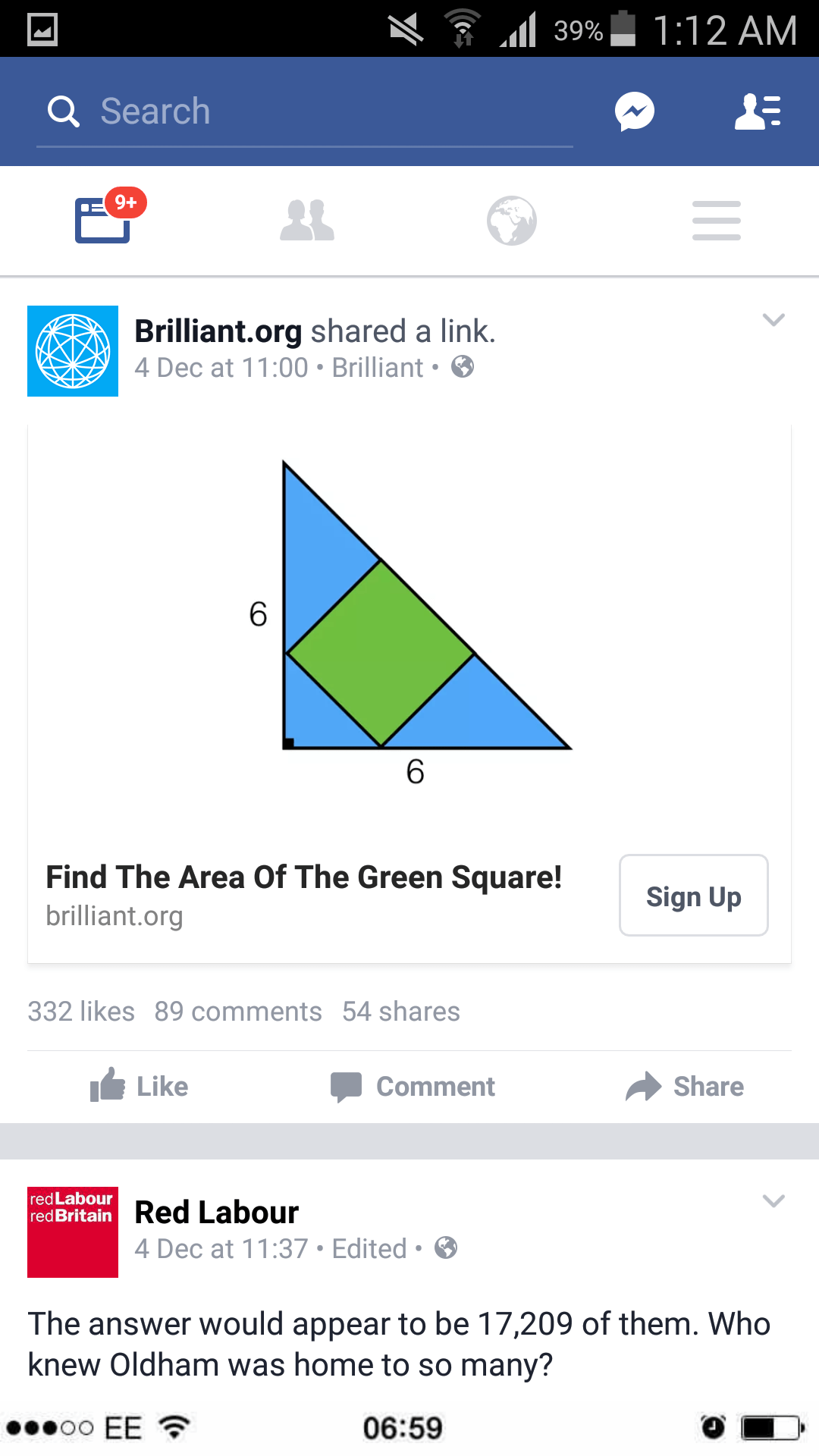Here is a post looking at a solution to, and possible extensions of, this puzzle: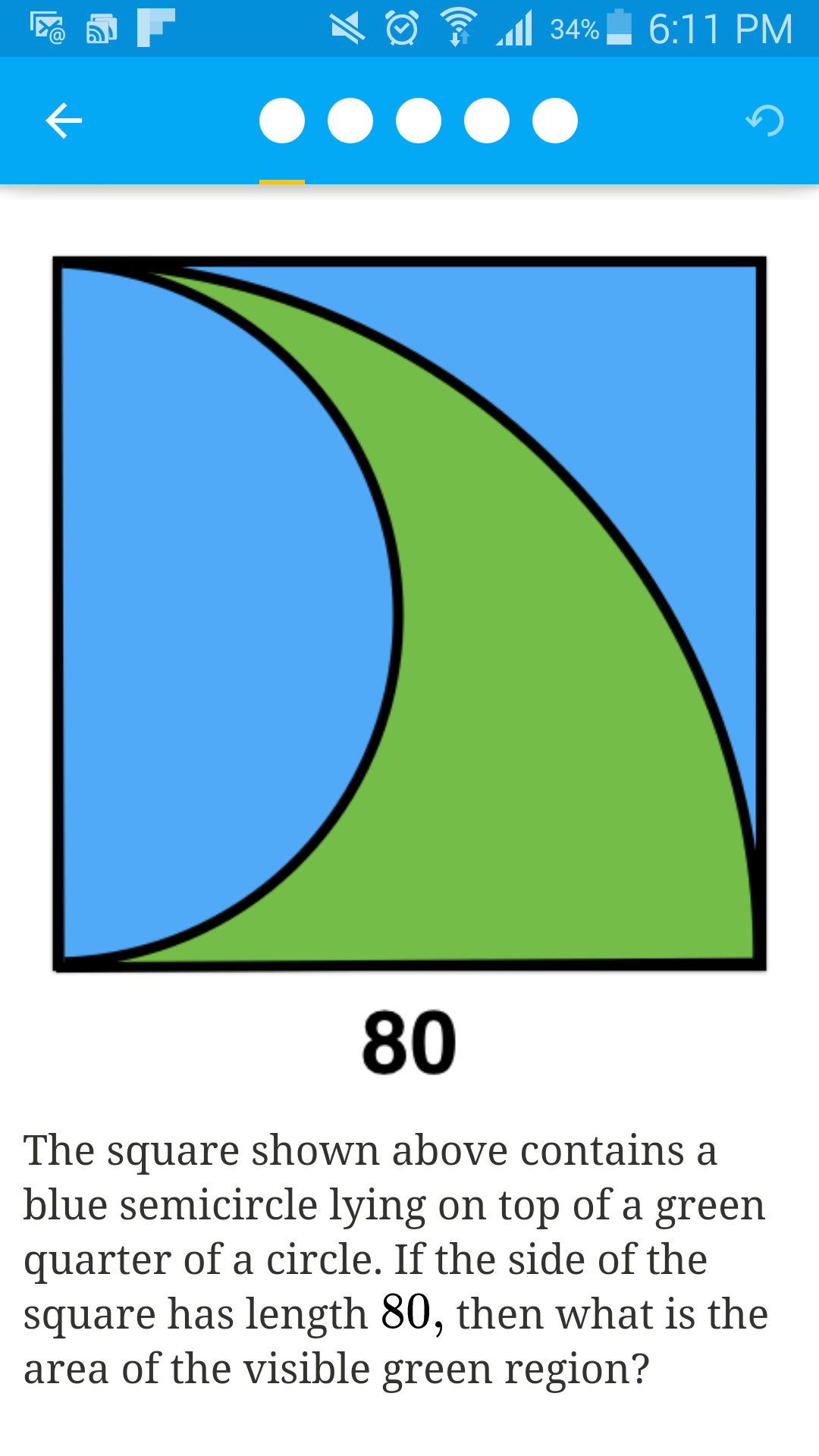4 Pics 1 word

A Venn Diagram puzzle

The mathematics concept wall

An old favourite SSM puzzle

Similarity, and other stories A look at similar triangles, Trigonometry, Pythagoras’s Theorem and area scale factors.

This is cool: An equilateral triangle and a regular hexagon have equal perimeter. The area of the triangle is 2 square units, what is the area of the hexagon. Solution at the end of this post.

“Wedding Maths” – a puzzle I discovered at a wedding!

The following are better suited to A Level students:

This lovely summation puzzle:A Probability Puzzle: “Colin and Dave are playing a game. Colin has a probability of 0.2 of hitting the target with any given shot; Dave has a probability of 0.3. Whoever hits the target first, wins. Colin goes first; what is his probability of winning?”

An acute problem: Prove that for an acute angled triangle ABC sinA + sin B + sin C > 2.

A Fiendishly Hard Puzzle: Use the numbers 1, 2, and 3 (in any order, exactly once each) and any mathematical symbols you like, to make 19.

A Cuboid Puzzleand a superb fractional puzzle: xy=3 x+y=2 what is 1/x + 1/y?

This page is also worth checking out for these kinds of puzzle, as is Emma Bell’s (@El_Timbre) page here. And Wrong, But useful have one per podcast!

I hope you enjoy these puzzles, and if you know of any please do let me know!